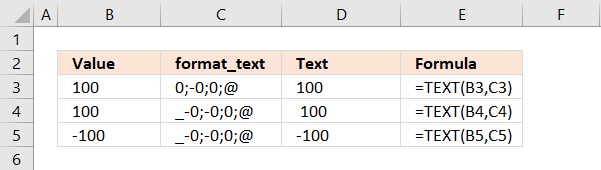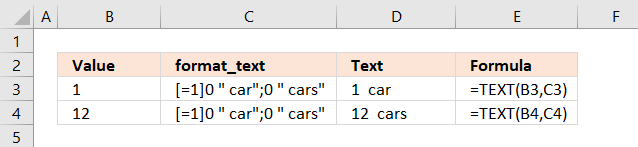Author: Oscar Cronquist Article last updated on June 16, 2018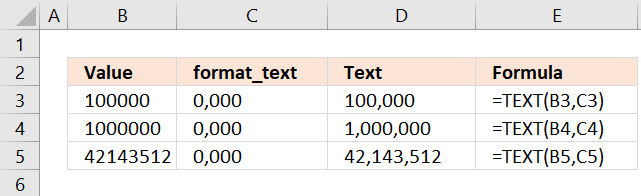### Excel function syntax

TEXT(value, format_text)

Converts a value to text in a specific number format.

### Arguments

value - The string you want to format. You can use a cell reference here or use a text string.

format_text - Formatting code allowing you to change the way, for example, a date or a number is displayed to the Excel user.

### Formatting basics

The TEXT function lets you easily create your own custom formats, there are four parts shown in the picture below.

You can use the semicolon to separate different settings for different values, the zero is a character symbol (among others) for numbers and the @ is a character symbol for text values.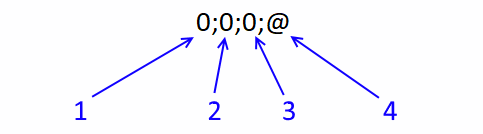1. Positive values
2. Negative values
3. Zero values
4. Text values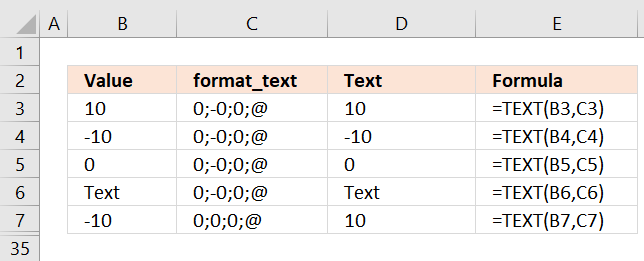You can ignore the semicolons if you want to format all values equal.

### Formatting codes you can use

. (period) - Decimal point. All digits to the left of the decimal point are shown no matter how many formatting characters.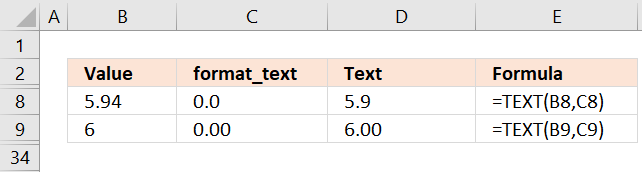, (comma) - The comma separates numbers into three characters. This makes large numbers easier to read.0 - Digit symbol. Example, type 4.5 in a cell and the format_text argument is 0.00 then the output from the TEXT function is 4.50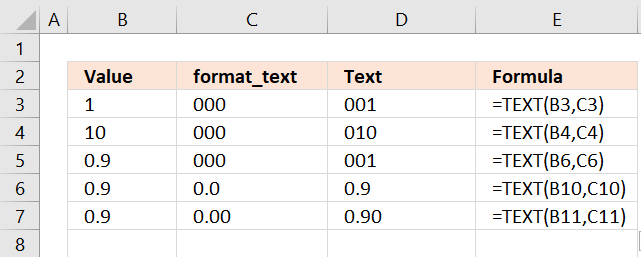# - Digit symbol. Does not show extra zeros. Example, type 4.5 in a cell and the format_text argument is #.## then the output from the TEXT function is 4.5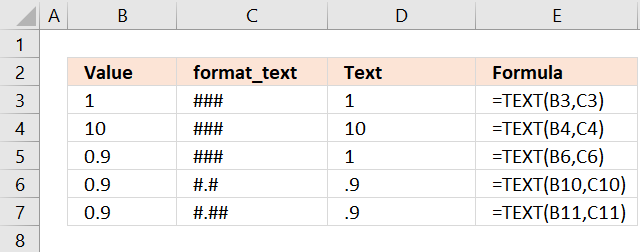? - Digit symbol, same as # but aligns the decimal points in a column.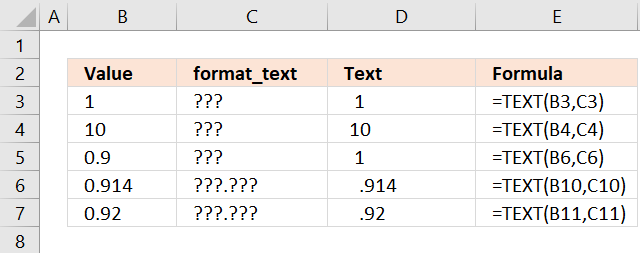% - Percentage. The percentage symbol multiplies the value with 100.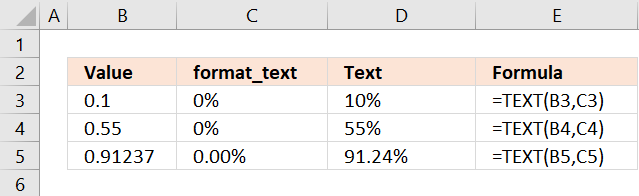E+ E- e+ e- - Scientific format.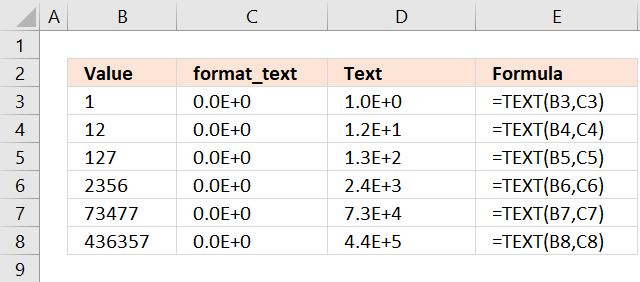@ - Text characters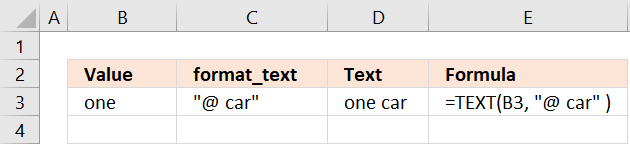"Text" - Characters enclosed by quotation marks shows the characters.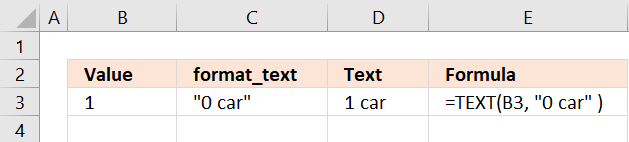m - Month number without a leading zero

mm - Month number with a leading zero when necessary

mmm - Month as an abbreviation, Jan, Feb, Mar, Apr, May, Jun, Jul, Aug, Sep, Oct, Nov and Dec.

mmmm - Full month name.

d- Day as a number without a leading zero

dd - Day as a number with a leading zero when necessary

ddd - Day as an abbreviation, Sun, Mon, Tue, Wed, Thu, Fri and Sat.

dddd - Full day name.

yy - Year as a two-digit number.

yyyy - Year as a four-digit number.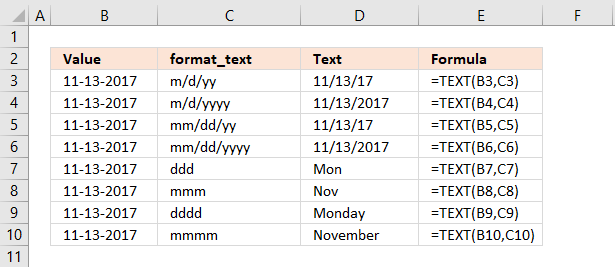h - Hour as a digit without a leading zero.

[h] - Shows more than 24 hours.

hh - Hour as a digit with a leading zero when needed.

m - Minute without a leading zero.

[m] - Shows more than 60 minutes.

mm - Minute with a leading zero when necessary.

s - Second without a leading zero.

[s] - Shows more than 60 seconds.

ss - Seconds with a leading zero when necessary.

AM/PM - Shows the time of 12-hour hours.

am/pm - See above.

A/P - See above.

a/p - See above.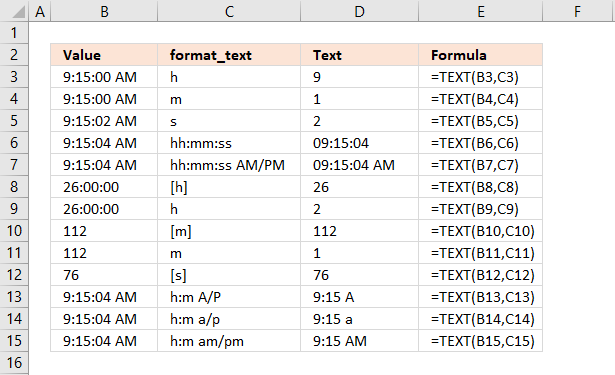Note! Check your regional settings if the format codes above don't work for you. Win 10 users can open the Control Panel and then click on "Regional settings" to view your settings.

#### Round numbers

The number of digit symbols you use after the decimal determines which number you want to round.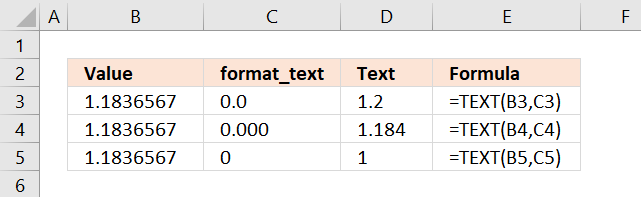You can round to thousands and millions and so on using the comma character.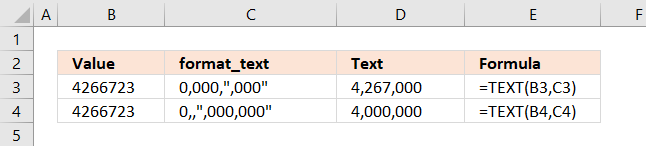#### Thousands and millions

The comma symbol scales the number by a thousand, two comma symbols scale the number by a million (1000 * 1000 = 1,000,000).

K = 1,000 and M = 1,000,000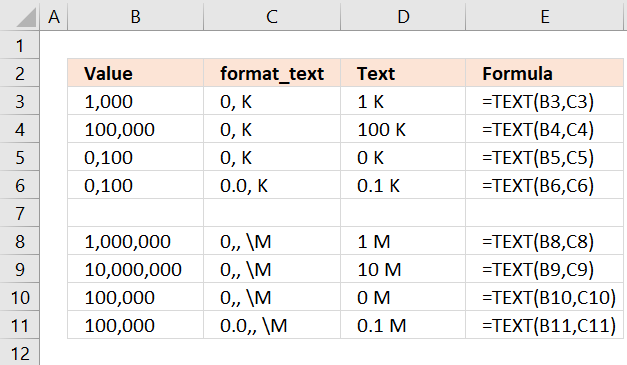The \ (backslash) symbol escapes the M character so Excel displays the letter M instead of converting the number into minutes.

The 0 (zero) symbol allows you to show leading zeros.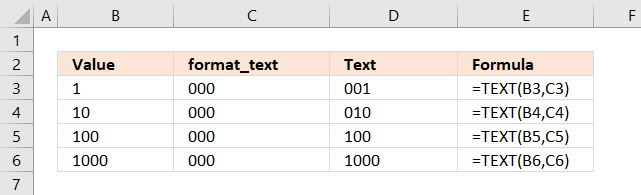#### Format positive, negative and zero values differently

The semicolon character lets you specify formatting code for positive, negative, zero values and text values. The picture below shows you these settings: 0.0;-0.00;"Zero";@

The first position is formatting code for positive values, here I have chosen 0.0. All positive values are rounded to one decimal.

The second position is affecting negative values, in this case, all negative values are rounded to two decimals.

The third position changes 0 to Zero and the fourth position shows the text value.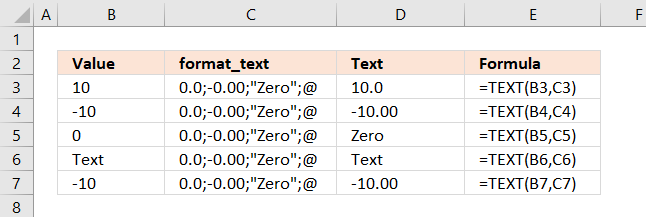#### Hide values

Positive values are hidden if you leave out the first position.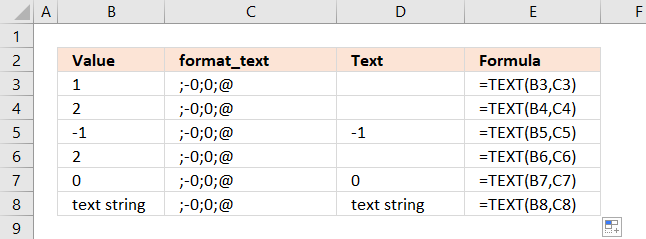Negative values are hidden if you leave the second position empty.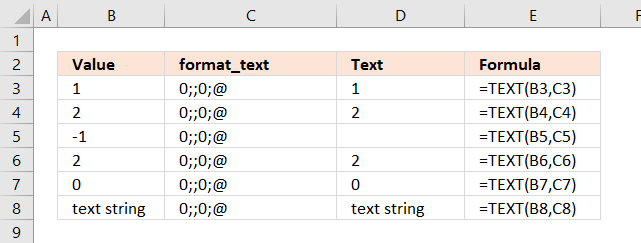If you leave the third position empty zeros are hidden.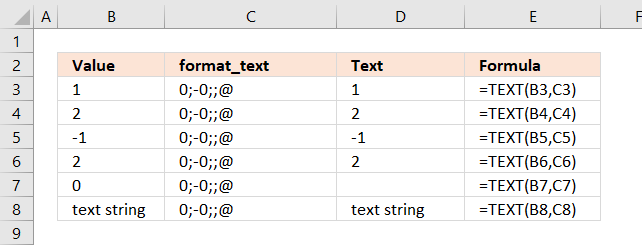Omit the fourth position to hide text values.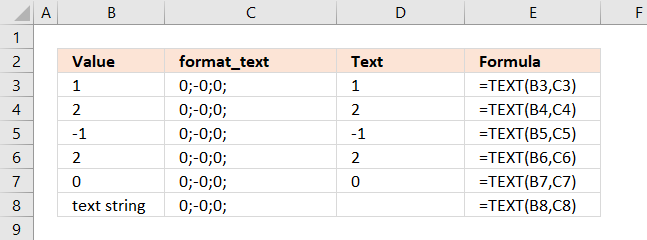#### Custom groups

Group 3 is assigned to values larger than 150, Group 2 is assigned to values larger than 100, Group 1 is assigned to all other values.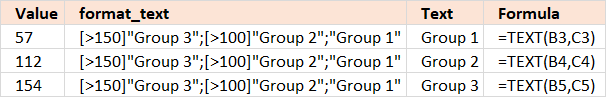#### Fractions

The division symbol lets you create fractions.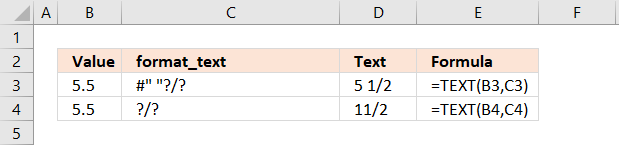#### Phone numbers

The 0 (zero) symbol lets you create phone numbers with leading 0's.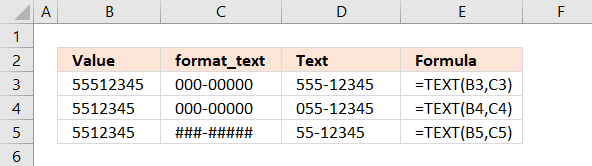#### Indents

Use the underscore character _ and then the character you want to use as an indent. The character is not shown.

The example below uses - (minus) as the indent character in the first position (positive numbers) to align negative and positive numbers. The first row is not aligned.